## 5.2.1 Hypotheses Testing

### Hypothesis Testing

Hypothesis Testing is a five-step procedure using sample evidence and probability theory to determine whether the hypothesis is a reasonable statement.

In other words, it is a method to prove whether or not the results obtained on a randomly drawn sample are projectable to the whole population.

Procedure:

1.State null and alternative hypothesis

2.Select a level of significance

3.Identify the test statistic

4.Formulate a decision rule

5.Take a sample, arrive at a decision

People are ‘erroneously confident’ in their knowledge and underestimate the odds that their information or beliefs will be proved wrong. They tend to seek additional information in ways that confirm what they already believed.”

Max Bazerman

Sex and Internet Usage

 Sex Internet usage Male Female Row total light 5 10 15 heavy 10 5 15 Column total 15 15 n=30

Based on this sample:

Q: Are there really more heavy internet users among males than among females in the general population?

### Step 1: State null and alternative hypothesis

A null hypothesis (H0)  is a statement of status quo, one of no difference or no effect.

An alternative hypothesis (H1) is one in which some difference or effect is expected.

H0:   There is no difference between males and females w.r.t.   internet usage.

#### IUm=IUf

H1:   Males and females expose different internet usage   behavior.

### Step 2: Select a level of significance

Significance (α) – probability of rejecting a true null hypothesis.

βprobability of accepting a false null hypothesis.

 Null hypothesis (H0) is true Null hypothesis (H0) is false Reject null hypothesis Type I error False positive Correct outcome True positive Fail to reject null hypothesis Correct outcome True negative Type II error False negative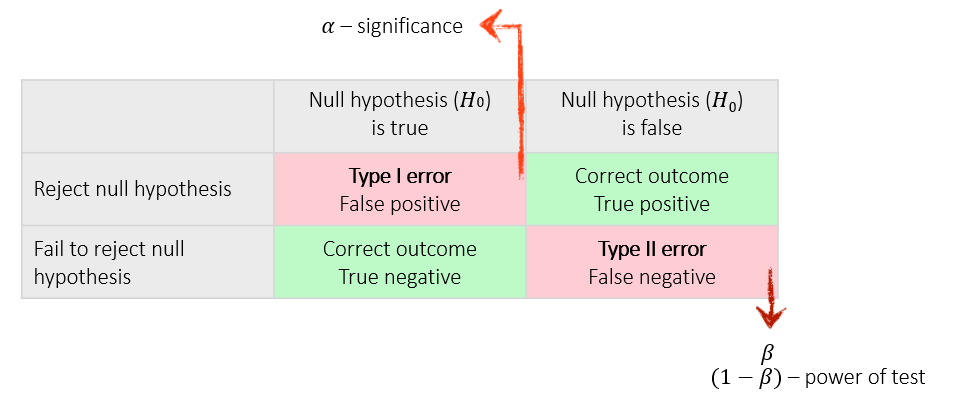Analogy: innocence in a criminal trial
H0: the defendant is innocent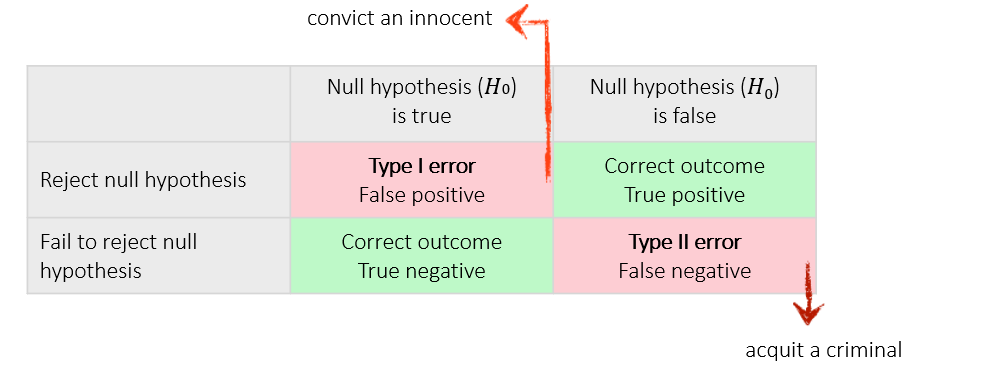Analogy: Rustle in the bush – is it a lion?
H0: there is no lion in the bush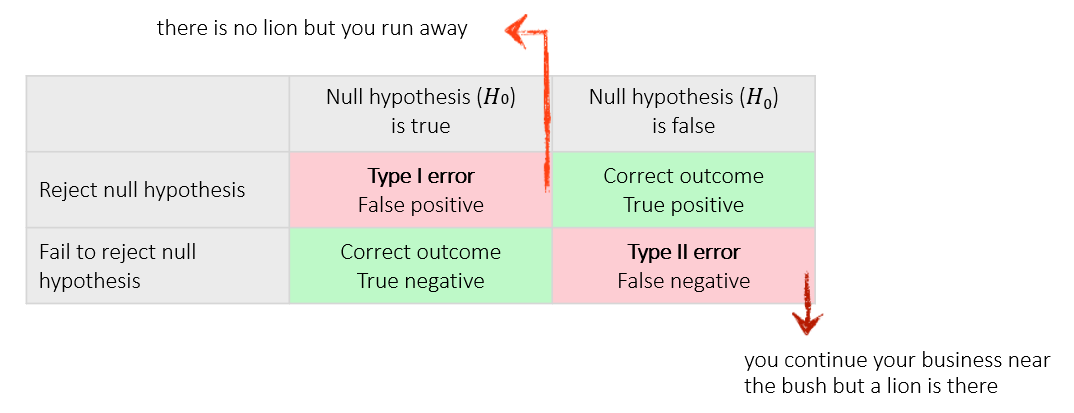Levels of significance in marketing research

α – level of significance

0.01 (1%)

0.05 (5%)

(1-α) – level of confidence

0.99 (99%)

0.95 (95%)

### Step 3: Identify the test statistic

 Sample Application Level of scaling Test/Comments One Sample Distributions Non-metric Kolmogorow-Smirnow and χ2 test for goodness of fit; Runs test for randomness; Binomial test for goodness of fit of dichotomous variables Means Metric t test, if variance is unknown z test, if variance is known Proportions Metric z test Two Independent Samples Distributions Non-metric Kolmogorow-Smirnow two-sample test for equality of two distributions Means Metric Two-group t test F test for equality of variances Proportions Metric Non-metric z test χ2 test Ranking/Medians Non-metric Mann-Whitney U test is more powerful than the median test Paired Samples Means Metric paired t test Proportions Non-metric McNemar test for binary variables, χ2 test Ranking/Medians Non-metric Wilcoxon matched-pairs ranked-signs test is more powerful than the sign test

χ2 (chi-square) statistic for goodness of fit is used to test the statistical significance of the observed association in a cross-tabulation

H0: There is no association between the variables

χ2 (chi-square) tests the equality of frequency distributions.
Which distributions/frequencies should we test?

fe – cell frequencies that would be expected if no association were present   between the variables

fo – actual observed cell frequencies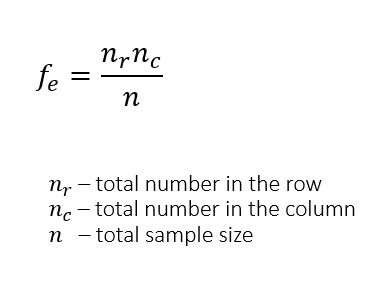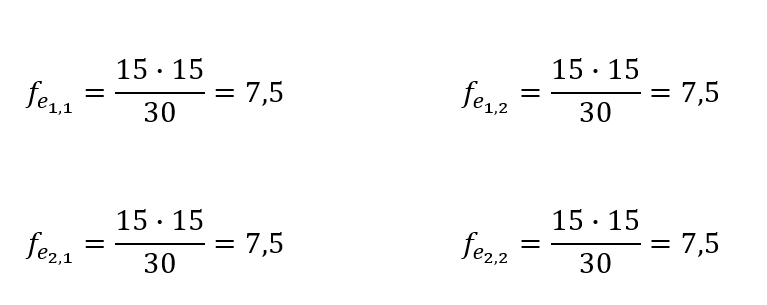χ2 statistic should be estimated only on counts of data. When the data are in percentage form, they should be first converted to absolute values or numbers.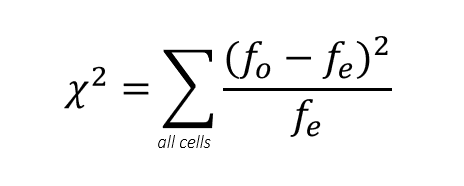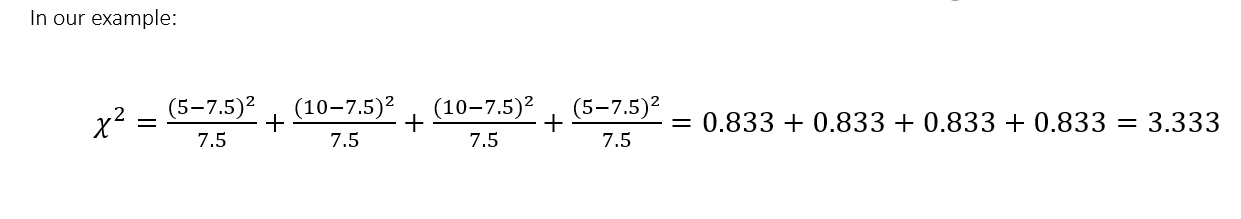### Step 4: Formulate a decision rule

TScal – observed value of the test statistic.

TScr critical value of the test statistic for a given significance level.

If probability of TScal < significance level (α), then reject H0.

or

If  TScal > TScr  , then reject H0.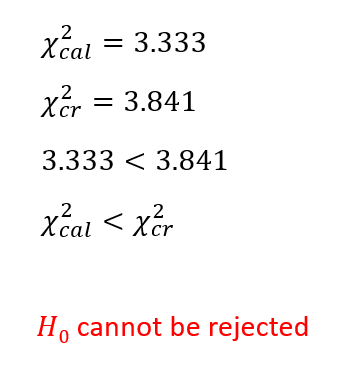Table of critical values of χ2 for different levels of significance α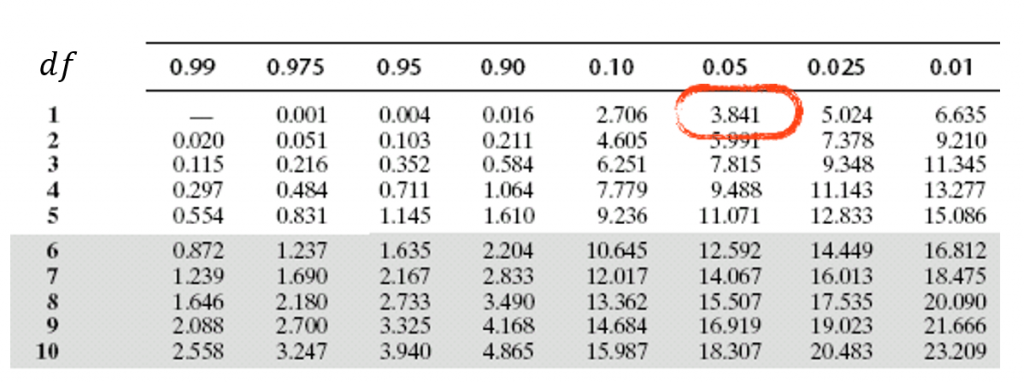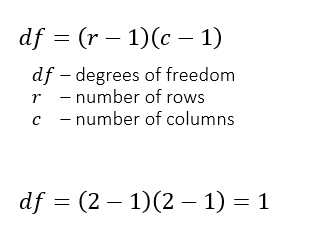### Step 5: Arrive at a decision

Is the evidence there?

What are the consequences?

• H0 of no association cannot be rejected
• Association is not statistically significant at the .05 level
• The findings from the sample cannot be generalized to population

Sex and Internet Usage

 Sex Internet usage Male Female Row total light 5 10 15 heavy 10 5 15 Column total 15 15 n=30

Based on this sample:

Q: Are there really more heavy internet users among males than among females in the general population?

A: The sample doesn’t provide such evidence.

If the sample was chosen and drawn appropriately, then we can state that there is no such relationship in the population at the 95% confidence level.
Otherwise – we don’t know.